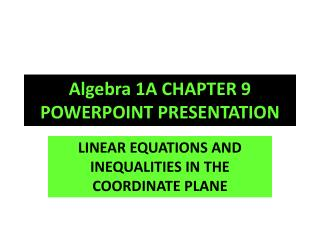DownloadDownload PresentationAlgebra 1 A CHAPTER 9 POWERPOINT PRESENTATION

# Algebra 1 A CHAPTER 9 POWERPOINT PRESENTATION

Download Presentation## Algebra 1 A CHAPTER 9 POWERPOINT PRESENTATION

- - - - - - - - - - - - - - - - - - - - - - - - - - - E N D - - - - - - - - - - - - - - - - - - - - - - - - - - -
##### Presentation Transcript

1. Algebra 1A CHAPTER 9 POWERPOINT PRESENTATION LINEAR EQUATIONS AND INEQUALITIES IN THE COORDINATE PLANE

2. LEARNING TARGETS • AFTER YOU COMPLETE THIS CHAPTER, YOU WILL BE ABLE TO: • IDENTIFY PARTS OF A GRAPH • LOCATE AND PLOT POINTS ON THE GRAPH • SOLVE EQUATIONS TO FIND AN ORDERER PAIR • DETERMINE THE SLOPE OF A GRAPH • SOLVE LINEAR EQUATIONS AND INEQUALITIES AND GRAPH THEM • INTERPRET GRAPHS

3. THE COORDINATE SYSTEM • x axis – runs horizontally • y axis – runs vertically • Origin – where x and y axis cross (0,0) • Quadrant – four sections of the graph, numbered with Roman Numerals, runs counter-clockwise • Ordered Pair – one x point and one y point (2,3) – can be plotted on the graph

4. SOME VISUAL EXAMPLES • THE GRID SYSTEM

5. Locating Points

6. GRAPHING • FIRST YOU MUST COMPLETE AN (X/Y TABLE) WITH AT LEAST THREE VALUES. • THEN YOU FIND THE COORDINATES AND PLACE THEM ON THE GRAPH. • YOU CONNECT THE COORDINATES TO FORM A STRAIGHT LINE. THAT IS A LINEAR EQUATION. • THE NEXT SLIDE CONTAINS AN EXAMPLE OF AN (XY TABLE AND THE GRAPH).

7. (x/y table and graph)

8. Intercepts • The x-intercept is where the line of the graph crosses the x- axis. • The y-intercept is where the line of the graph crosses the y-axis.

9. SLOPE • SLOPE IS THE MEASURE OF THE STEEPNESS OF THE LINE ON THE GRAPH. THERE ARE FOUR KINDS: • POSITIVE • NEGATIVE • SLOPE OF ZERO • UNDEFINED SLOPE

10. POSITIVE SLOPE

11. NEGATIVE SLOPE

12. SLOPE OF ZERO

13. UNDEFINDED SLOPE

14. LINEAR EQUATION • THE EQUATION FOR A GRAPH WITH A STRAIGHT LINE: y = mx + b • m = the slope • b = the y-intercept

15. VOCABULARY • DEPENDENT VARIABLE – THE VALUE OF THE Y VARIABLE THAT DEPENDS ON THE VALUE GIVEN FOR X. • INDEPENDENT VARIABLE – THE VALUE OF X WHICH DETERMINES THE VALUE OF Y • FUNCTION – A RULE THAT STATES THERE IS ONLY ONE X VALUE FOR EACH Y VALUE • VERTICAL LINE TEST – A VERTICAL LINE INTERSECTS THE GRAPH

16. VERTICAL LINE TEST

17. MORE VERTICAL LINE TEST

18. DOMAIN AND RANGE OF A FUNCTION • FUNCTION – ONE AND ONLY ONE X VALUE • RELATION – MORE THAN ONE X VALUE FOR EACH Y VALUE • DOMAIN – ALL THE X VALUE • RANGE – ALL THE Y VALUES

19. INEQUALITIES • USE THE FOLLOWING SYMBOLS:

20. GRAPHS OF INEQUALITES

21. OTHER GRAPHS OF INEQUALITIES

22. GRAPHS OF OTHER FUNCTIONS

23. SLOPE FORMULA